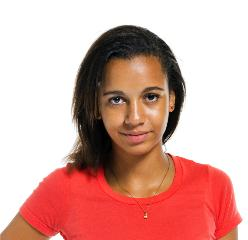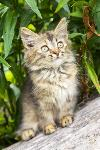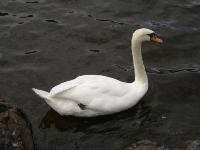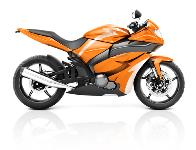# 18.10. Write Code Exercises¶

The picture below are used in the following exercises.

Data file: `gal2.jpg`Data file: `kitten.jpg`Data file: `swan.jpg`Data file: `motorcycle.jpg`Data file: `baby.jpg`Data file: `guy1.jpg`Note

Remember that it can take a bit of time to process all the pixels in a picture! Check for errors below the code if it is taking a long time, but if you don’t see any errors just wait.

1. Fix 4 syntax errors in the code below so that it correctly sets the red in all pixels to 0.

2. The code below makes the image have a green-blue tint. Change 1 thing in order to make it have a red tint instead.

3. Fix the indention below to correctly set the red to the green, the green to the blue, and the blue to the red.

4. Fix the 5 errors in the code, so that the Red pixels get the value of the green, the green get the value of blue, and the blue get the value of the red. (The cat should look purple and gray)

5. Fill in the missing code on lines 9, 12, and 18 below to set the red to half the original value in all pixels in the picture.

6. Complete the code in order to set the blue value to an eighth of the green value plus an eighth of the red value.

7. Fix the indention in the code below so that it correctly increases the red in each pixel in the picture by 1.5.

8. This code is supposed to make the picture completely black; however, it is taking forever when it should only take a few seconds. Fix the code (without adding anything new) so that it runs in a few seconds.

9. Fix the code below to correctly set the green and blue values to 0.75 times their current values.

10. The code below sets all the pixels to half their original values with one for loop. Change the code so it uses 2 for loops that utilize the range function (1 for loop should be nested in the other).

11. Change the following code to set the red to 0 for all pixels in the left half of the picture.

12. The code below makes the whole image have a blue-green tint. Change the code so that it makes an only blue tint in the bottom left corner.

13. Change the code below to set the red value in the pixels in the bottom half of the picture to 0.

14. The code below makes the whole image seem red. Change it, so that only every 5 pixels get changed, so that it will look like a red grid.

15. Change the following code into a procedure to keep only the green values in all pixels in a picture.

16. A grayscale picture is when the red, green, and blue value of a pixel are all equal to the average of the original pixel value. Write the code to turn the left half of an image into gray scale.

17. Define a procedure to negate an image. You can negate an image by setting each color value to 255 minus the current value.

18. Write code that copies the top half of an image to the bottom half.

19. Write a function to mirror an image from left to right around a vertical line in the middle of the image. Pass the image to the function. Do the import, create the image, call the function, and show the result.

20. Write code that flips the image across a horizontal line.

21. Create a subclass of the Image class with three different image effect functions and use each effect on a different part of the image. For example, you could turn the image into grayscale, keep only the green values, swap the red and green values, negate the image, etc. For example, the image shown below has one image effect on the first third of the image (negate), one on the second third (clear blue), and one on the final third (reduce red). You will also need to create an __init__ method and a main function that creates an object of your class and calls the three functions on that object. Be sure to call the main function. You can use any of the images shown in chapter 18 like arch.jpg or beach.jpg.